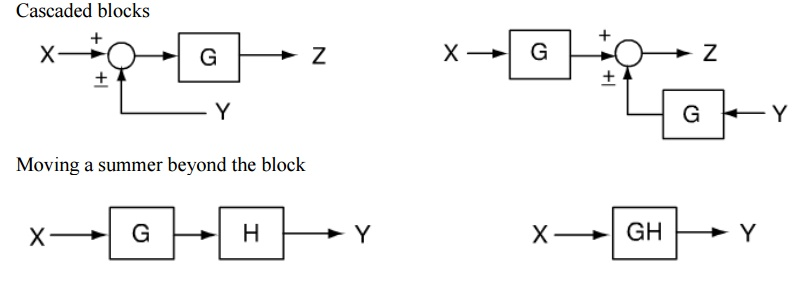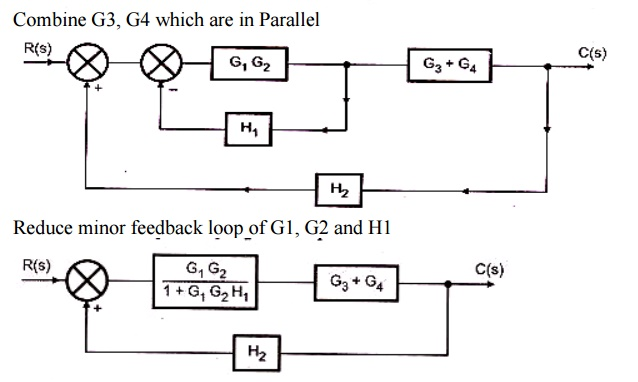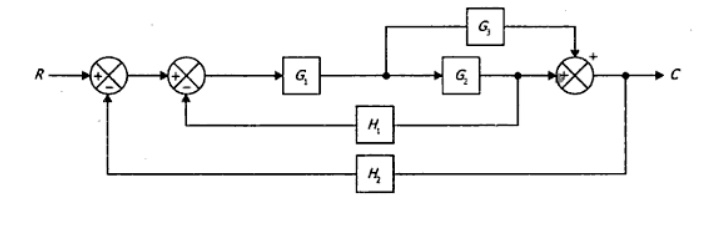Home | | Control System Engineering | Block diagram reduction Techniques - Transfer Function

# Block diagram reduction Techniques - Transfer Function

A pictorial representation of the functions performed by each component and of the flow of signals.

Block diagram

A pictorial representation of the functions performed by each component and of the flow of signals.

Basic elements of a block diagram

o   Blocks

o   Transfer functions of elements inside the blocks

o   Summing points

o   Take off points

o   Arrow

Block diagram

A control system may consist of a number of components. A block diagram of a system is a pictorial representation of the functions performed by each component and of the flow of signals.

The elements of a block diagram are block, branch point and summing point.

Block

In a block diagram all system variables are linked to each other through functional blocks. The functional block or simply block is a symbol for the mathematical operation on the input signal to the block that produces the output.Summing point

Although blocks are used to identify many types of mathematical operations, operations of addition and subtraction are represented by a circle, called a summing point. As shown in Figure a summing point may have one or several inputs. Each input has its own appropriate plus or minus sign.

A summing point has only one output and is equal to the algebraic sum of the inputs.A takeoff point is used to allow a signal to be used by more than one block or summing point. The transfer function is given inside the block

The input in this case is E(s)

The output in this case is C(s)

C(s) = G(s) E(s)Functional block  each element of the practical system represented by block with its T.F.

Branches  lines showing the connection between the blocks

Arrow  associated with each branch to indicate the direction of flow of signal

Closed loop system

Summing point  comparing the different signals

Take off point  point from which signal is taken for feed back

o   Very simple to construct block diagram for a complicated system

o   Function of individual element can be visualized

o   Individual & Overall performance can be studied

o   Over all transfer function can be calculated easily.

o   No information about the physical construction

o   Source of energy is not shown

Simple or Canonical form of closed loop systemR(s) – Laplace of reference input r(t)

C(s) – Laplace of controlled output c(t)

E(s) – Laplace of error signal e(t)

B(s) – Laplace of feed back signal b(t)

G(s) – Forward path transfer function

H(s) – Feed back path transfer function

Block diagram reduction technique

Because of their simplicity and versatility, block diagrams are often used by control engineers to describe all types of systems. A block diagram can be used simply to represent the composition and interconnection of a system. Also, it can be used, together with transfer functions, to represent the cause-and-effect relationships throughout the system. Transfer Function is defined as the relationship between an input signal and an output signal to a device.

Block diagram rulesProcedure to solve Block Diagram Reduction Problems

Step 1: Reduce the blocks connected in series Step

2: Reduce the blocks connected in parallel Step 3: Reduce the minor feedback loops

Step 4: Try to shift take off points towards right and Summing point towards left

Step 5: Repeat steps 1 to 4 till simple form is obtained

Step 6: Obtain the Transfer Function of Overall System

Problem 1

1. Obtain the Transfer function of the given block diagram2. Obtain the transfer function for the system shown in the fig3. Obtain the transfer function C/R for the block diagram shown in the figSolution

The take-off point is shifted after the block G2Study Material, Lecturing Notes, Assignment, Reference, Wiki description explanation, brief detail
Control Systems : Systems and their Representation : Block diagram reduction Techniques - Transfer Function |